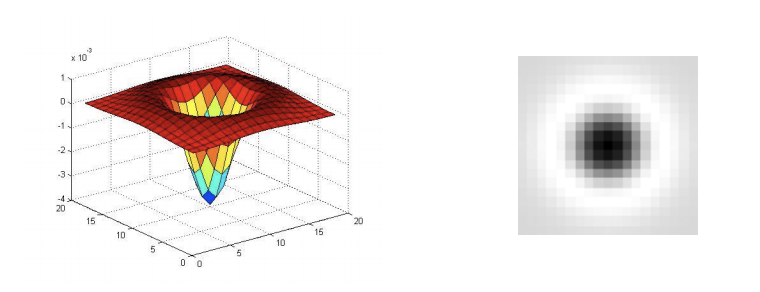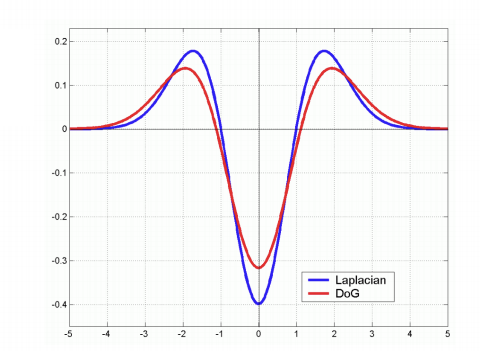# “Feature detection 2”

## Image transformation

1. Geometric: Rotation, Scale
2. Photometric: Intensity change

## Invariance and equivariance

We want corner locations to be invariant to photometric transformations and equivariant to geometric transformations

1. Invariance: image is transformed and corner locations do not change
2. Equavariance: if we have two transformed versions of the same image, features should be detected in corresponding locations

## Some operartion and their characteristic

1. Derivatives and window function are equivariant
2. Corner location is equivariant w.r.t. translation
3. Corner location is equivariant w.r.t. image rotation
4. Affine intensity change: Partially invariant to affine intensity change
5. Scaling is neither invariant nor equivariant to scaling

## Key idea to scale invariant

find scale that gives local maximum of f

1. in both position and scale
2. one definition of f: the Harris operator
3. Instead of computing f for larger and larger windows, we can implement using a fixed window size with a Gaussian pyramid

## Laplacian of Gaussian$\nabla^{2} g=\frac{\partial^{2} g}{\partial x^{2}}+\frac{\partial^{2} g}{\partial y^{2}}$

This is a “Blob” detector, the maxima and minima of LoG operator in space and scale are blobs in the image

## An alternative

DoG: Difference of Gaussians[D o G=G(x, y, k \sigma)-G(x, y, \sigma)]## YONG HUANG

Hi, I'm Yong Huang. I've recently graduated from Cornell Tech and obtained my master's degree, I shall start my Ph.D. in Computer Science this fall at UC Irvine. Thank you for visiting my site.